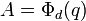# Every finite division ring is a field

Jump to: navigation, search

## History

This result was first proved by Wedderburn.

## Statement

Every finite division ring is a field, and hence, a finite field. Here, by division ring, we mean associative division ring.

## Proof

This proof uses a tabular format for presentation. Provide feedback on tabular proof formats in a survey (opens in new window/tab) | Learn more about tabular proof formats|View all pages on facts with proofs in tabular format

Given: A division ring$K$ of finite size.

To prove:$K$ is a field.

Proof': We denote by$K^*$ the multiplicative group of nonzero elements of$K$.

The key idea behind the proof is to switch between the additive/linear structure and the multiplicative structure. For the additive structure, we use key fact that the size of a vector space over a field is a power of the size of the field. For the multiplicative structure, we use Lagrange's theorem (a multiplicative constraint) and the class equation (a combinatorial constraint on how things should add up). Finally, we show that the number theory does not work out.

Step no. Assertion/construction Facts used Given data used Previous steps used Explanation Commentary
1 Let$L$ be the center of$K$, i.e., the subset of$K$ comprising those elements that commute multiplicatively with every element of$K$.
2$L$ is a field under the operations induced from$K$, and the multiplicative group$L^*$ of nonzero elements of$L$ is the center of$K^*$.$K$ is a division ring. [SHOW MORE] Creating base field for which many of the additive structures of interest are vector spaces.
3$K$ is a vector space over$L$ where the additive structure is the same as that for$K$ as a skew field and where the action of elements of$L$ is by left multiplication. Step (2) [SHOW MORE] Linear consolidation over$L$.
4 If$L$ has size$q > 1$,$K$ has size$q^d$ for some positive integer$d$, where$d$ is the dimension of$K$ as a vector space over$L$.$K$ is finite Step (3) [SHOW MORE] Linear consolidation over$L$, introduce numerical constraints. Note that$q$ must in fact be a prime power, but we only need use here that$q > 1$.
5 For an element$g \in K$, define$C_K(g)$ to be the set of elements of$K$ that commute with$g$. Then,$C_K(g)$ is a subspace of$K$ as a$L$-vector space. It contains$L$ and has dimension$r$ and size$q^r$ for$1 \le r \le d$. Further, if$g \notin L$, then$1 < r < d$ ($r$ depends on$g$). Step (4) [SHOW MORE] Linear consolidation over$L$.
6 For$g \in K \setminus L$, the centralizer of$g$ in the multiplicative group$K^*$ has size$q^r - 1$ for$1 < r < d$ ($r$ depends on$g$) Step (5) For the multiplicative group, we need to throw out the zero element. Convert linear/additive constraint to constraint on multiplicative group.
7 The class equation of$G$ is:$\! q^d - 1 = (q - 1) + \sum_{i=1}^s \frac{q^d - 1}{q^{r_i} - 1}$ where$r_1, r_2, \dots, r_s$ are dimensions over$L$ of the centralizers in$K$ of representatives of conjugacy classes in$K^*$ of non-central elements. For each$i$, we have$1 < r_i < d$. Fact (2) Steps (2) -- (6) This is a direct application of the construction of the class equation. Combinatorial constraint on multiplicative group.
8 Each$r_i$ divides$d$. Fact (1) Steps (2), (3), (6) [SHOW MORE] Combine linearly arising multiplicative constraints with basic number theory to get further constraints.
9 Let$A = \Phi_d(q)$ where$\Phi_d$ is the cyclotomic polynomial with parameter$d$. Then, as an integer,$A$ divides$q^d - 1$ as well as each of the quotient$(q^d - 1)/(q^{r_i} - 1)$ appearing in step (7). Steps (7), (8) [SHOW MORE] Pure cyclotomic polynomial theory (number theory/algebra)
10 If$d > 1$, then$A = \Phi_d(q)$ does not divide$q - 1$ [SHOW MORE] Pure cyclotomic polynomial theory (number theory/algebra)
11 We can conclude$d = 1$, so$K = L$ and$K$ is a field. Steps (7), (9), (10) [SHOW MORE] Show irreconciliability of constraints derived from linear structure and constraints arising from cyclotomic polynomial theory.